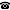LCF abstracts

Borodulin-Nadzieja, Piotr: Geometry of analytic P-ideals

We say that an ideal on $\omega$ is represented in a Banach space $X$ if there is a sequence $(x_n)_n$ in $X$ such that $A$ belongs to the ideal if and only if $\sum_{n\in A} x_n$ is unconditionally convergent. This is a generalization of the notion of summability: an ideal is represented in $\mathbb{R}$ if and only if it is summable. We pose several questions on which ideals are represented in certain Banach spaces and we answer some of them. We show that this approach reveals some geometric properties of analytic P-ideals and has a potency of generating new examples of ideals on $\omega$.

This is a joint work with Barnabás Farkas and Grzegorz Plebanek.

Holy, Peter: Locally $\Sigma_1$-definable Wellorders of $H(\kappa^+)$

We show that if $\kappa$ is uncountable with $\kappa^{<\kappa}=\kappa$, it is consistent to have a $\Sigma_1$-definable wellorder of $H(\kappa^+)$ and $2^\kappa$ large. This contrasts the case $\kappa=\omega$, where Mansfield showed that a $\Sigma_1$-definable wellorder of $H(\omega_1)$ implies CH.

This is joint work with Philipp Luecke.

Ikegami, Daisuke: Inner models from logics and the generic multiverse

The goal of this research is to construct a model of set theory which is "close to" HOD but easier to analyze. The motivation comes from Woodin's HOD Conjecture, which states that HOD is very "close to" V under the presence of a very strong large cardinal (extendible cardinal). HOD Conjecture is closely related to the problem of constructing a canonical extender model with a supercompact cardinal and it has striking applications to the theory of large cardinals without the Axiom of Choice.

To solve HOD Conjecture, one would expect a fine analysis of HOD. The difficulty of the analysis of HOD lies in the fact that HOD is very "non-absolute", e.g., one could force V = HOD with a proper class partial order.

Given that HOD is obtained using full second order logic in the same way as Gödel's constructible universe L via first order logic, in this talk, we use Boolean valued higher order logics and Woodin's $\Omega$-logic to construct inner models of set theory which are more "absolute" than HOD and investigate the properties of the models.

Koszmider, Piotr: The Banach space $C(N*)$ in the Cohen model.

We will present results of a joint paper with C. Brech: http://arxiv.org/abs/1211.3173 concerning the consistency of some properties of the Banach space $C(N^*)\equiv\ell_\infty/c_0$ obtained in the Cohen model. We will also mention some of many open problems concerning this Banach spaces induced by the algebra $P(N)/Fin$ which could depend on extra set-theoretic assumptions.

Mejía, Diego: Rothberger gaps in $F_\sigma$ ideals

We investigate a cardinal invariant associated with gaps of a given $F_\sigma$-ideal of $\omega$, which we call the Rothberger number of the ideal. We concentrate on the class of fragmented ideals and prove that it is consistent to obtain infinitely many different Rothberger numbers associated to such ideals. Also, we present some examples of fragmented ideals with Rothberger number equal to $\aleph_1$.

Mildenberger, Heike: Finitely Many Near-Coherence Classes of Ultrafilters

I will describe a recent forcing construction that establishes for any finite number $n\geq 2$ that it is consistent relative to ZFC that there are eactly $n$ near coherence classes of ultrafilters. This settles the old question on the possible numbers of near coherence classes: any finite non-zero number or $2^c$ are the only possibilities, and for each of them we have a model. The main new technique is to destroy selective ultrafilters by a forcing in a way that they can be complemented to selective ultrafilters in the forcing extension.

Rinot, Assaf: Hedetniemi’s conjecture for uncountable graphs

It is proved that if $V=L$, then for every successor cardinal κ, there exist graphs $G$ and $H$ of size and chromatic number κ, for which the tensor product graph $G×H$ is countably chromatic. This solves a longstanding open problem of Hajnal, and establishes the consistency of the failure of the infinite weak Hedetniemi conjecture.

Sargsyan, Grigor: On the strength of the unique branch hypothesis (UBH)

We show that certain failures of UBH imply the existence of a non-tame mouse. This significantly improves the previous lower bound due to Steel.

This is a joint work with Nam Trang.

Schindler, Ralf: Does $\Pi^1_1$ determinacy yield $0^\#$?

It is unknown whether $\Pi^1_1$ determinacy yields $0^\#$ in 3rd order arithmetic. We show that 3rd order arithemtic plus Harrington's principle "there is a real $x$ such that every $x$-admissible is an $L$-cardinal" is equiconsistent with ZFC plus a remarkable cardinal, and we also discuss strengthenings of Harrington's principle.

This is joint work with Cheng Yong.

Kurt Gödel Research Center for Mathematical Logic. Währinger Straße 25, 1090 Wien, Austria.+43-1-4277-50501. Last updated: 2013-09-22, 23:48.